## A handy calculator with steps to solve linear and quadratic equations# Quadratic Equation Solver with Steps

by Intemodino Group s.r.o.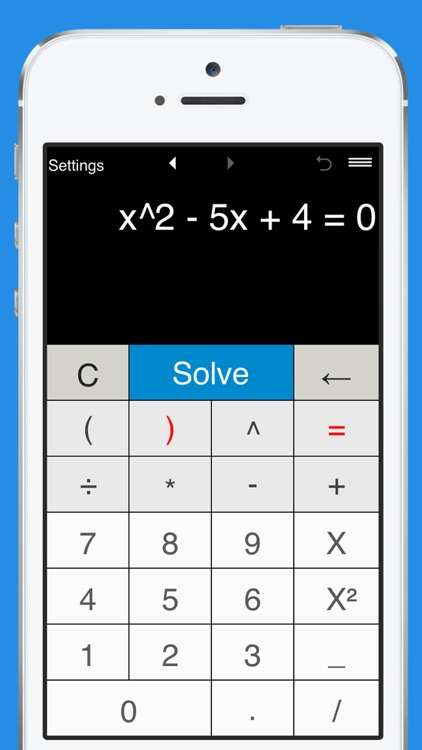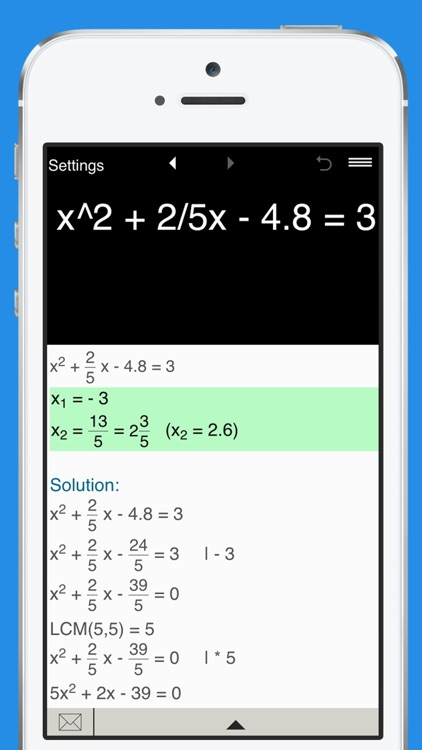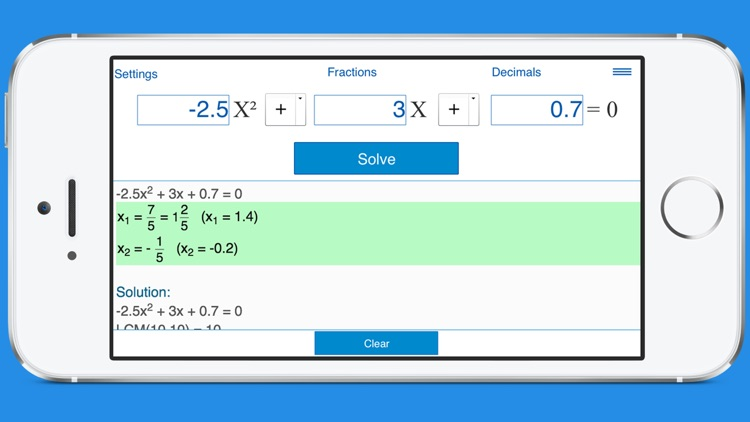A handy calculator with steps to solve linear and quadratic equations.### App Details

Version
2.0.3
Rating
(5)
Size
23Mb
Genre
Education Utilities
Last updated
February 12, 2016
Release date
November 11, 2012

### App Screenshots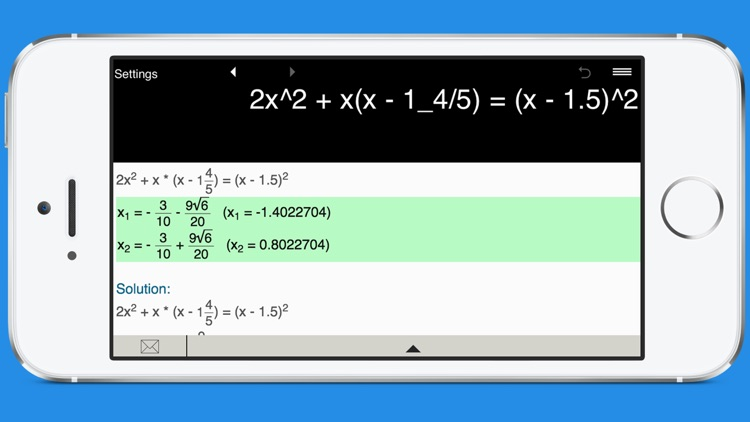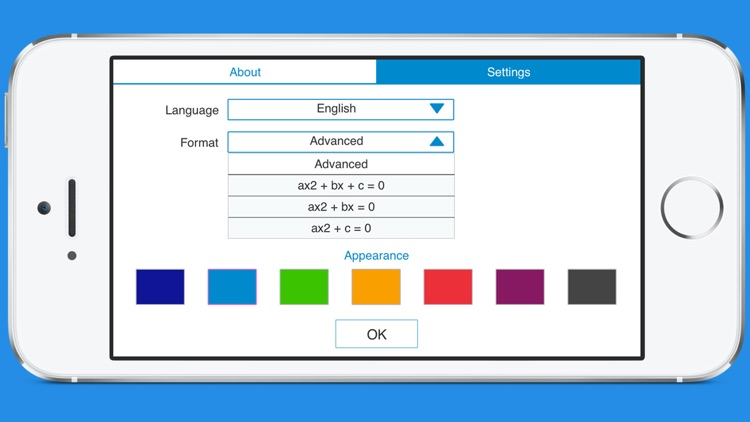### App Store Description

A handy calculator with steps to solve linear and quadratic equations.

Quadratic Equation Solver can handle a wide range of linear and quadratic equations, including equations with fractions and parentheses.

Features:

Solves quadratic equations with real and complex roots.

Solves linear equations.

Shows step-by-step solution.

Supports fractions and parentheses. Note: The calculator does not solve equations with a variable in the denominator.

Pre-defined formats to solve quadratic equations in the form ax^2 + bx + c = 0, ax^2 + bx = 0 and ax^2 + c = 0. Just enter the values of a, b and c, and click Solve. You can enter integer, fractional and decimal coefficients.

Stores history with the possibility to recall previous equations.

Sends results and history via email.

Disclaimer:
AppAdvice does not own this application and only provides images and links contained in the iTunes Search API, to help our users find the best apps to download. If you are the developer of this app and would like your information removed, please send a request to takedown@appadvice.com and your information will be removed.